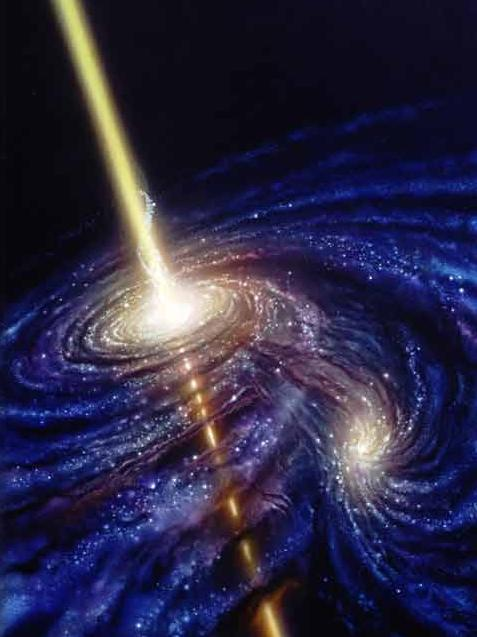# Black Holes

In Black Holes by Brian Koberlein1 Comment

The basic idea of a black hole is simple. Imagine tossing a ball into the air. It goes up to a certain height, and then back down. If you toss the ball faster, the ball rises higher, but it still eventually falls. Now suppose you could toss the ball as fast as you like. Could you toss the ball so fast it doesn’t fall back down?

The answer is yes. For any mass, there is a speed that is large enough to escape its gravitational pull. That minimum escape speed is known as the escape velocity. For the Earth (ignoring air resistance) the escape velocity is about 11 kilometers per second, or about 34 times the speed of sound. Typically smaller masses have smaller escape velocities and larger masses larger ones. For example Pluto has an escape velocity a bit larger than 1 km/s, while the Sun’s escape velocity is more than 600 km/s.

But there is another way to get a higher escape velocity, and that is to have a more dense mass. If you packed the same amount of mass into a smaller volume, then the gravity on its surface is larger, and it takes a greater speed to get away. For example if the Earth had the same mass, but half its actual radius, then its escape velocity would be about 16 km/s.

Suppose then that you could take the mass of a star or planet and pack it into as small of a volume as you like. What would happen? The smaller the volume you packed your mass into, the larger the escape velocity. If you kept packing it into a smaller and smaller volume, you would reach a point where the escape velocity is equal to the speed of light. Pack it into an even smaller size, and you’d have to travel faster than light to escape. Not even light would be fast enough to escape your mass. You would have made a black hole.

The speed of light is 300,000 km/s, which is huge for an escape velocity. That means your mass would need to be packed into a very small volume. To make the Earth into a black hole you would have to squeeze it down to the size of a marble. Even a black hole with the mass of the Sun would have a diameter of less than 6 kilometers (about 3.5 miles).

Of course this simple idea uses Newton’s idea of gravity. To describe a real black hole we need to use Einstein’s theory, specifically the idea that gravity is a curvature of space. If you do the math in Einstein’s gravity, you find that a real (non-rotating) black hole is exactly the size predicted by Newton’s gravity.

But since gravity is a curvature of space and time, things are a little bit different. Gravity is a curvature of space and time. Far away from a black hole, spacetime is only curved slightly, and objects are attracted only slightly, just like regular masses. As you get closer to the black hole, spacetime is curved more strongly. Even closer and you reach a point where space and time are curved so strongly they trap anything inside it. The surface of this trap is known as the event horizon of the black hole, and that event horizon is located exactly where the escape velocity is the speed of light.

What this means is that from far away a black hole pulls on you just like any large mass would. Just like any mass, the closer you get the more strongly it will pull you, and the harder it will be to escape its gravity. But if you get too close, if you cross the boundary known as the event horizon, you will find yourself trapped. There is no way for you to escape. Space and time are curved so tightly that not even light can escape. Cross the event horizon and you can never leave.

Essentially a black hole is a cosmic roach motel.

1.